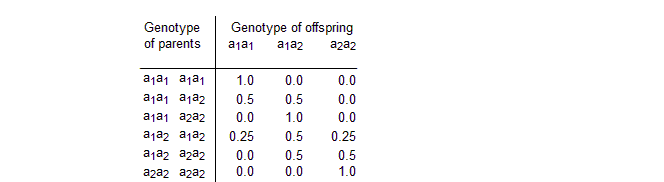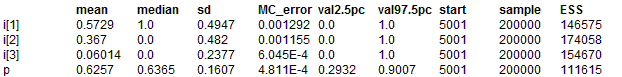#### Pigs: genetic counseling and pedigree analysis

Spiegelhalter (1990) uses exact methods to analyse a small pedigree. This pedigree was previously used by Cannings and Thompson (1981) to illustrate their 'peeling' procedure to provide likelihoods for gene frequencies and probabilities for individuals being affected or carriers. We assume the pedigree refers to pigs which have the possibility of carrying a recessive gene: thus each pig has a genotype a1a1, a1a2 or a2a2, in which only those with a2a2 are affected with the trait, while those with a1a2 are carriers of the defective allele a2. We assume that Ian (the consequence of a mating between Fred and his Aunt Clare) is yet to be born, and all that is known is that Fred's niece Jane has the trait. We wish to estimate the prevalence p of the allele a2, and predict the chance of Ian being affected. The conditional probability distributions are as follows. For the genotype of the founder nodes Ann, Brian, Eric and Henry we assume a binomial distribution

Founder   ~   Binomial(q, 2)

where Founder takes values 0, 1 or 2 for genotypes a2a2, a1a2 and a1a1 respectively, and q = 1- p is the prevalence of the allele a1. This is equivalent to assuming Hardy Weinberg equilibrium, giving P(a1a1) = q2, P(a1a2) =2 q (1- q) , P(a2 a2) = (1- q)2.

For the genotype of offspring we have the standard Mendelian inheritance probabilities given by the following table.For a recessive gene the genotype-to-phenotype penetrance probabilities are given by:The necessary inheritance probabilities are read in from the data file as an array

BUGS code for Pigs model:
model
{
q ~ dunif(0,1) # prevalence of a1
p <- 1 - q # prevalence of a2
Ann1 ~ dbin(q,2); Ann <- Ann1 + 1 # geno. dist. for founder
Brian1 ~ dbin(q,2); Brian <- Brian1 + 1
Clare ~ dcat(p.mendelian[Ann,Brian,]) # geno. dist. for child
Diane ~ dcat(p.mendelian[Ann,Brian,])
Eric1 ~ dbin(q,2)
Eric <- Eric1 + 1
Fred ~ dcat(p.mendelian[Diane,Eric,])
Gene ~ dcat(p.mendelian[Diane,Eric,])
Henry1 ~ dbin(q,2)
Henry <- Henry1 + 1
Ian ~ dcat(p.mendelian[Clare,Fred,])
Jane ~ dcat(p.mendelian[Gene,Henry,])
A1 ~ dcat(p.recessive[Ann,]) # phenotype distribution
B1 ~ dcat(p.recessive[Brian,])
C1 ~ dcat(p.recessive[Clare,])
D1 ~ dcat(p.recessive[Diane,])
E1 ~ dcat(p.recessive[Eric,])
F1 ~ dcat(p.recessive[Fred,])
G1 ~ dcat(p.recessive[Gene,])
H1 ~ dcat(p.recessive[Henry,])
I1 ~ dcat(p.recessive[Ian,])
J1 ~ dcat(p.recessive[Jane,])
a <- equals(Ann, 2) # event that Ann is carrier
b <- equals(Brian, 2)
c <- equals(Clare, 2)
d <- equals(Diane, 2)
e <- equals(Eric, 2) ;
f <- equals(Fred, 2)
g <- equals(Gene, 2)
h <- equals(Henry, 2)
for (J in 1:3) {
i[J] <- equals(Ian, J) # i = a1 a1
# i = a1 a2
# i = a2 a2 (i.e. Ian affected)
}    }
##### Data
``` list(p.mendelian = structure(.Data=c(1.0, 0.0, 0.0,                     0.5, 0.5, 0.0,                     0.0, 1.0, 0.0,                      0.5, 0.5, 0.0,                     0.25, 0.5, 0.25,                     0.0, 0.5, 0.5,                     0.0, 1.0, 0.0,                         0.0, 0.5, 0.5,                     0.0, 0.0, 1.0), .Dim=c(3,3,3)),      p.recessive = structure(.Data=c(1.0, 0.0,                         1.0, 0.0,                        0.0, 1.0), .Dim=c(3,2)),                                 A1 = 1, B1 = 1, C1 = 1, D1 = 1, E1 = 1, F1 = 1, G1 = 1, H1 = 1, J1 = 2) ```
##### Inits for chain 1
``` list(Ann1 = 2, Brian1 = 2, Clare = 2, Diane = 2, Eric1 = 2, Fred = 2, Gene = 2, Henry1 = 2, Ian = 2, Jane = 3) ```

##### Inits for chain 2
``` list(Ann1 = 2.0,Brian1 = 1.0,Clare = 3.0,Diane = 2.0,Eric1 = 2.0,Fred = 3.0,Gene = 3.0,Henry1 = 2.0,I1 = 2.0,Ian = 3.0,Jane = 3.0,q = 0.7381) ```

Results
One update took 0.06ms

Update methods

Updater type   Size   Depth
Ann1   discrete slice updater   1   2
Brian1   discrete slice updater   1   2
Clare   discrete slice updater   1   3
Diane   discrete slice updater   1   3
Eric1   discrete slice updater   1   2
Fred   discrete slice updater   1   4
Gene   discrete slice updater   1   4
Henry1   discrete slice updater   1   2
I1   univariate forward updater   1   -6
Ian   univariate forward updater   1   -5
Jane   discrete slice updater   1   5
q   conjugate beta updater   1   1DIC direct parents crashes
We note a number of important tricks. First, each genotype is a 3-valued categorical variable with conditional probabilities either determined by the binomial (Hardy-Weinberg equilibrium) distribution (for founder nodes) or from the Mendelian inheritance probabilities which are stored as a 3-dimensional array p.mendelian. In the latter case, the genotype of the parents picks which row of the matrix is used for the distribution. However, the rows of this matrix are indexed by values 1, 2 or 3, whilst the genotypes of the founder nodes take values 0, 1 or 2. Since BUGS does not allow subscripts to be functions of variables, we must first add 1 to the genotype of the parents (for example, Ann = Ann1 + 1) and use these new variables as subscripts to the matrix p.mendelian. The genotype-to-phenotype distribution is handled similarly in a matrix p.recessive. Second, the equals function equals(Ann, 2) allows the calculation of P(Ann's genotype = 2) (i.e. a carrier), whilst equals(Ian, J) calculates P(Ian's genotype = J), where J=3 implies that Ian is affected.

Check of externalization first process

mean   median   sd   MC_error   val2.5pc   val97.5pc   start   sample   ESS
i   0.5717   1.0   0.4948   0.001695   0.0   1.0   5001   100000   85192
i   0.3685   0.0   0.4824   0.00153   0.0   1.0   5001   100000   99367
i   0.05981   0.0   0.2371   8.037E-4   0.0   1.0   5001   100000   87047
p   0.6258   0.636   0.1608   7.119E-4   0.2935   0.9013   5001   100000   51034

second process
mean   median   sd   MC_error   val2.5pc   val97.5pc   start   sample   ESS
i   0.5717   1.0   0.4948   0.001695   0.0   1.0   5001   100000   85192
i   0.3685   0.0   0.4824   0.00153   0.0   1.0   5001   100000   99367
i   0.05981   0.0   0.2371   8.037E-4   0.0   1.0   5001   100000   87047
p   0.6258   0.636   0.1608   7.119E-4   0.2935   0.9013   5001   100000   51034

mean   median   sd   MC_error   val2.5pc   val97.5pc   start   sample   ESS
i   0.5729   1.0   0.4947   0.001292   0.0   1.0   5001   200000   146575
i   0.367   0.0   0.482   0.001155   0.0   1.0   5001   200000   174058
i   0.06014   0.0   0.2377   6.045E-4   0.0   1.0   5001   200000   154670
p   0.6257   0.6365   0.1607   4.811E-4   0.2932   0.9007   5001   200000   111615